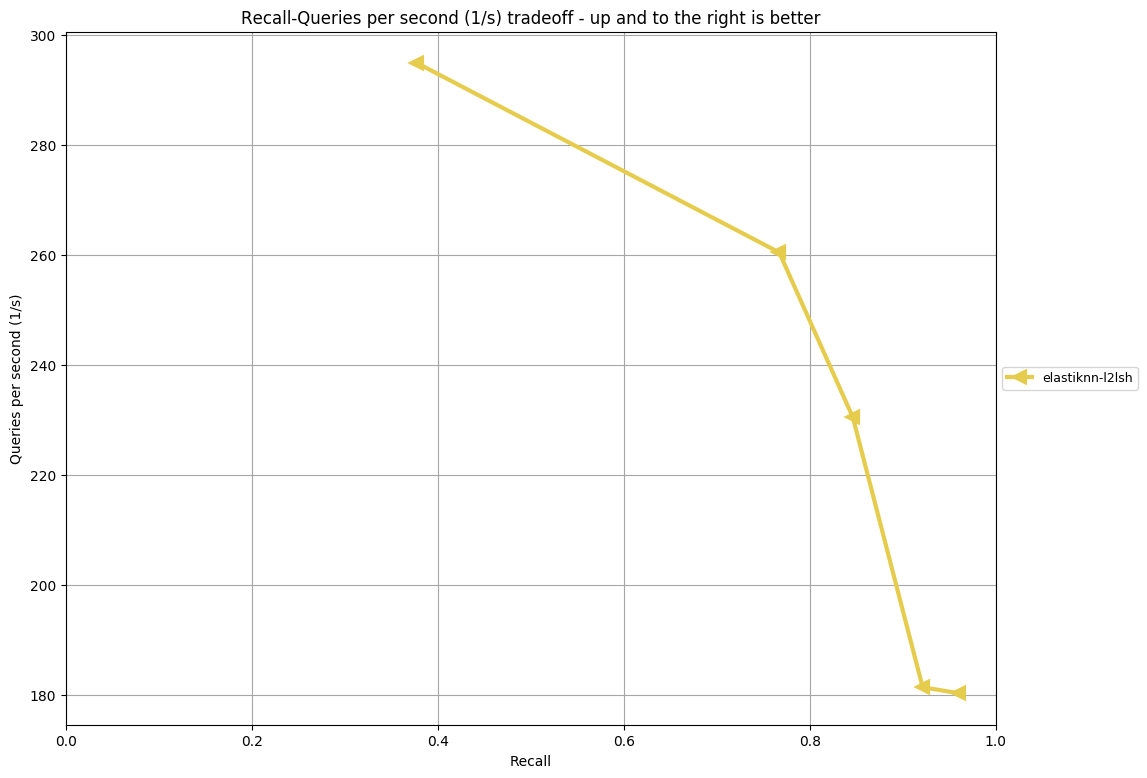# Performance

## Method

The results on this page are produced by running elastiknn on the ann-benchmarks benchmark on a 2018 Mac Mini (6-Core i7, 64GB RAM variant):

Caveats

• This is a single-node benchmark, so latency from communication across cluster nodes is eliminated.
• The client and server run in the same container, so latency from client/server communication is minimized.

See the ann-benchmarks directory in the Elastiknn repository for more details.

## Results

### Fashion MNISTModel Parameters Recall Queries per Second
eknn-l2lsh L=100 k=4 w=1024 candidates=500 probes=0 0.378 294.909
eknn-l2lsh L=100 k=4 w=1024 candidates=1000 probes=0 0.445 259.962
eknn-l2lsh L=100 k=4 w=1024 candidates=500 probes=3 0.634 238.267
eknn-l2lsh L=100 k=4 w=1024 candidates=1000 probes=3 0.716 210.426
eknn-l2lsh L=100 k=4 w=2048 candidates=500 probes=0 0.767 260.469
eknn-l2lsh L=100 k=4 w=2048 candidates=1000 probes=0 0.846 230.483
eknn-l2lsh L=100 k=4 w=2048 candidates=500 probes=3 0.921 181.336
eknn-l2lsh L=100 k=4 w=2048 candidates=1000 probes=3 0.960 180.251

## Comparison to Other Methods

If you need high-throughput nearest neighbor search for batch jobs, there are many faster methods. When comparing Elastiknn performance to these methods, consider the following:

1. Elastiknn executes entirely in the Elasticsearch JVM and is implemented with existing Elasticsearch and Lucene primitives. Many other methods use C and C++, which are generally faster than the JVM for pure number crunching tasks.
2. Elastiknn issues an HTTP request for every query, since a KNN query is just a standard Elasticsearch query. Most other methods operate without network I/O.
3. Elastiknn stores vectors on disk and uses zero caching beyond the caching that Lucene already implements. Most other methods operate entirely in memory.
4. Elastiknn scales horizontally out-of-the-box by adding shards to an Elasticsearch index. Query latency typically scales inversely with the number of shards, i.e., queries on an index with two shards will be 2x faster than an index with one shard. Few other methods are this simple to parallelize.